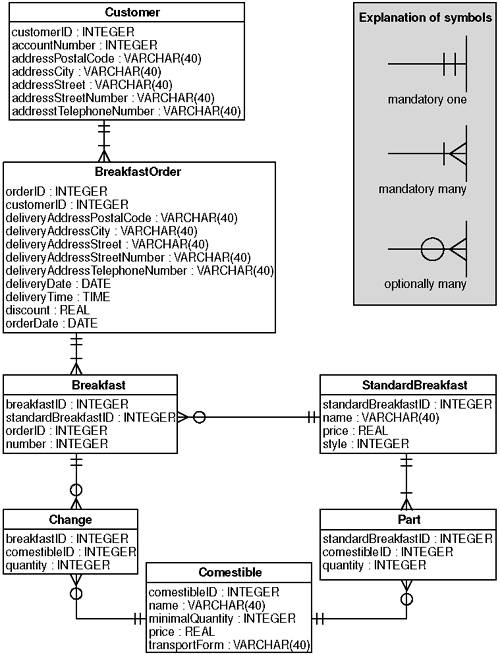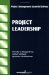# 5.1 The PIM to Relational Transformation

The transformation rules for generating relational database models typically take care of a consistent object-relational mapping. Although most of these rules are rather straightforward and well-known (Blaha 1998), it can be a hard job to execute them manually. Small changes in the PIM can have a large effect on the relational model. For instance, changing the type of an attribute in the PIM from a simple data type to a class means introducing a foreign key in the corresponding table. The simple data type can be mapped directly to a column in a table. But if the data type is a class, this class will be mapped to a table itself. The column will now have to hold a reference (foreign key) to a key value in that other table.

What rules should be used to generate a relational model? Note that we want to formulate rules that will apply to any UML model, not only to Rosa's PIM. First, we must decide how the basic data types are being mapped. This is a fairly simple task. All we need to do is find the right corresponding data type in the relational model. Data types can be mapped according to the following rules. Note that we define an arbitrary length for each of the relational data types.

• A UML string will be mapped onto a SQL VARCHAR(40).

• A UML integer will be mapped onto an INTEGER.

• A UML date will be mapped onto a DATE.

But what do we with the Address? In the PIM the address is not a class, but a data type, a struct containing only attributes, and no operations. We have two options: either make a separate table for every data type, or inline the data type into the table that holds the attribute. Here we choose the latter option, because it will simplify the alignment with the EJB model. So for struct data types we have the following rule:

• A UML data type that has no operations will be mapped onto a number of columns , each representing a field in the data type.

Second, every class should be transformed into a table, where all attributes are fields in the table (rules ClassToTable and AttrToCol). When the type of the attribute is not a data type but a class, the field in the table should hold a foreign key to the table representing that class (rule AttrToFrkey). Note that we do not yet take into account the possibility that the multiplicity of the attribute is more than one.

The third step is more complicated. Associations in the UML model need to be transformed into a foreign key relation in the database model, possibly introducing a new table. Note that we have several possibilities for the multiplicities of an association from class A to class B in a UML model:

• The multiplicity at A is zero-or-one.

• The multiplicity at A is one.

or

• The multiplicity at A is more than one.

The same holds for the multiplicity at B. This leaves us with nine different combinations of multiplicities at both ends. Furthermore, we have to take into account that an association can be adorned with an association class. The rule can best be expressed in pseudocode:

`  if  the association A to B is adorned by an association class    or the multiplicity at both ends is more-than-one  then  create a table representing the association class or the       association       and create foreign keys in both the table representing A and       the table representing B referring this new table  else if  the multiplicity at one end is zero-or-one  then  create a foreign key in the table representing the class          at that end, referencing the other end  else  /* the multiplicity of the association is one-to-one */          create a foreign key in one of the tables, referencing the          other end  endif   endif  `

Note that in this example we do not take into account the navigability of the association. We assume, for the sake of simplicity, that all associations are navigable.

Each column in a relational model may or may not have the NULL value. In this transformation, only the columns generated from the attributes of the PIM may have the value NULL. The other columns are generated based on the association ends to constitute the foreign keys. These columns may not have the value NULL. The following rules correspond with the above:

• A UML attribute will be mapped to a column that may contain the NULL value.

• A UML association end will be mapped to a number of columns that may not contain the NULL value.

Figure 5-1 depicts the resulting database model in the form of an Entity-Relationship diagram. You can see that there is one table for each class in the PIM. Columns that are part of the key are repeated as foreign key columns in tables representing the "many" side of the association. The struct called address is inlined into the Customer and BreakfastOrder tables, and for each field there is one column in the table. The fact that a column may have the NULL value is not shown in the diagram, but assumed to be part of the generated relational model.

##### Figure 5-1. Relational PSM of Rosa's Breakfast ServiceProject Leadership (The Project Management Essential Library)
ISBN: N/A
EAN: 2147483647
Year: 2004
Pages: 118

Similar book on Amazon